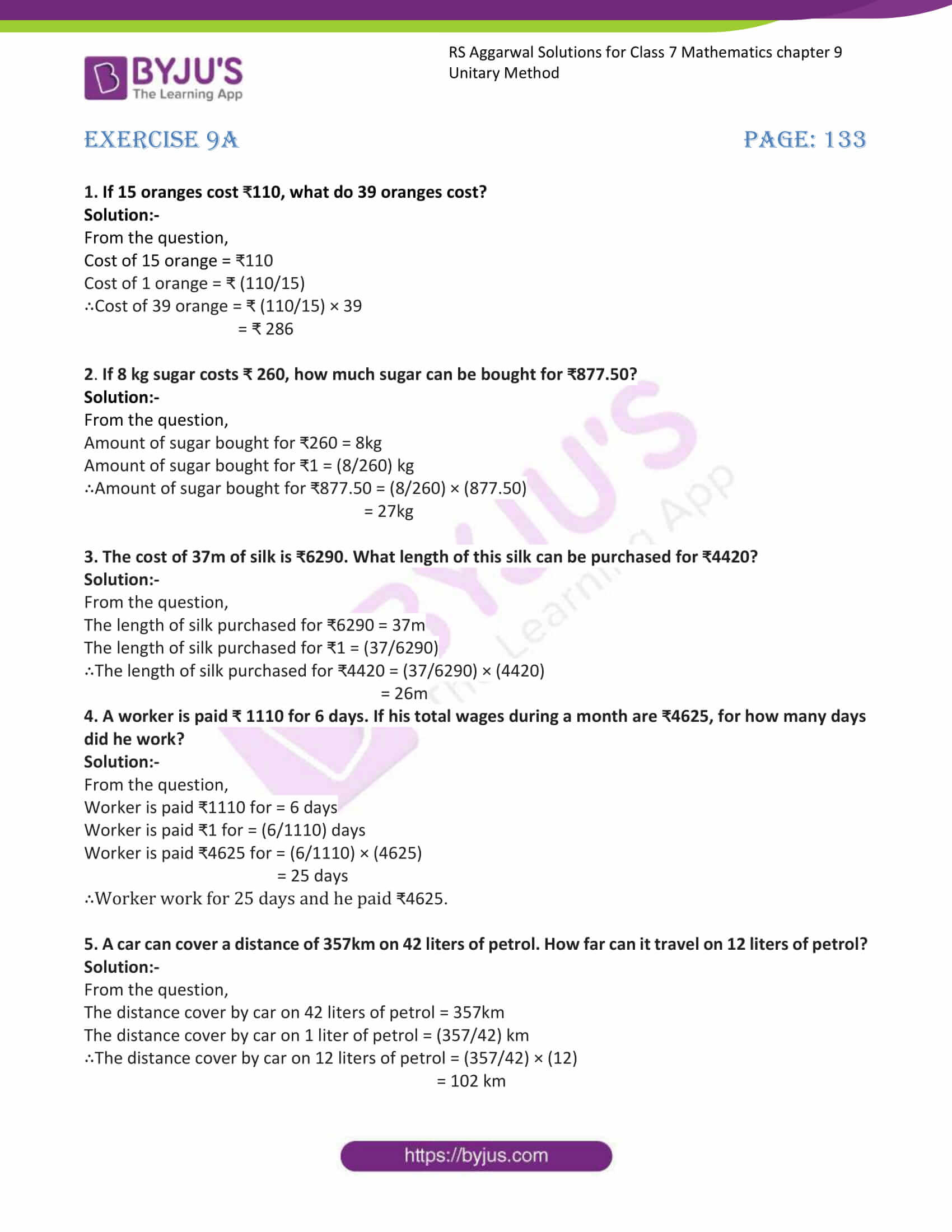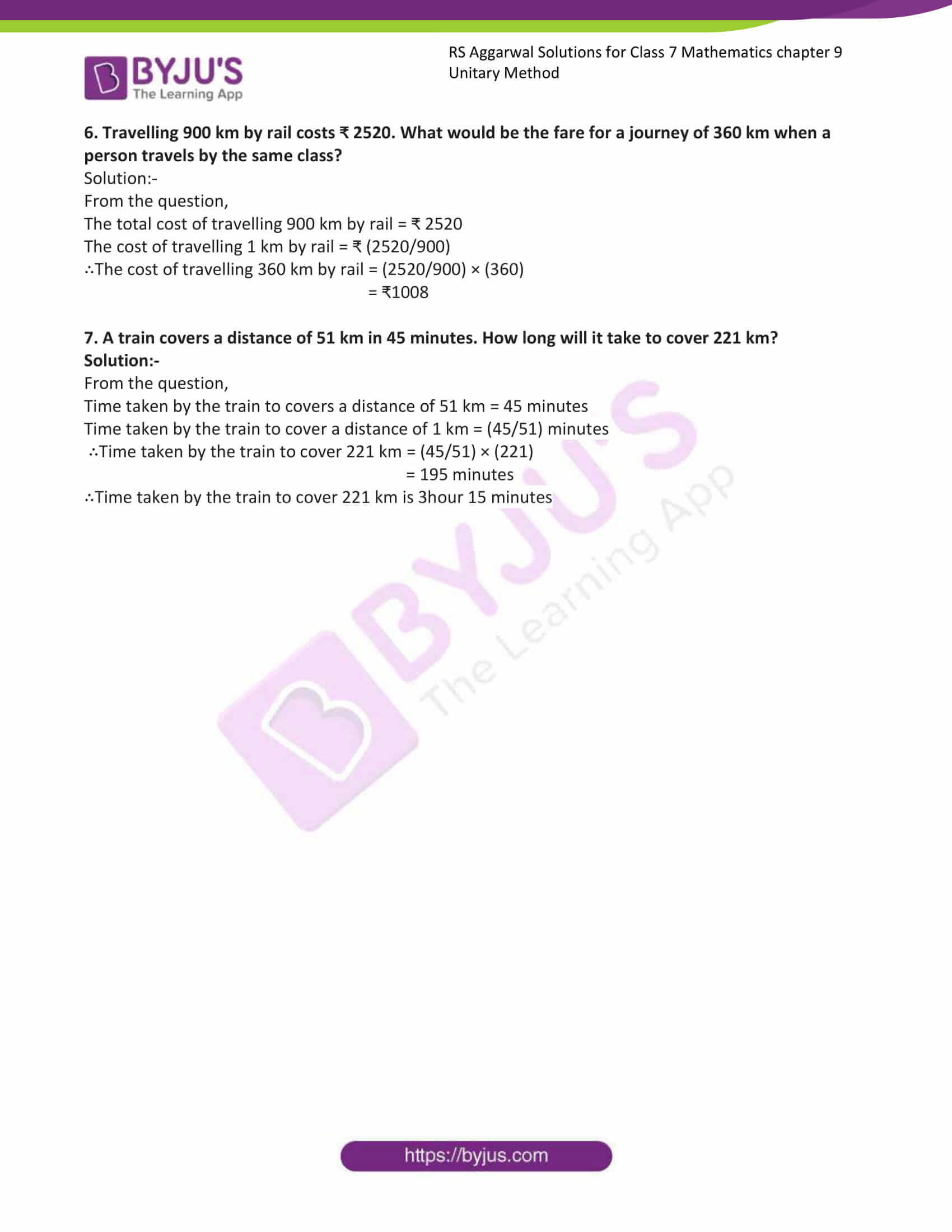# RS Aggarwal Solutions for Class 7 Maths Exercise 9A Chapter 9 Unitary Method

The simple PDF of RS Aggarwal Solutions for the Exercise 9A of Class 7 Maths Chapter 9, Unitary Method is the best study material for those students who are finding complications in solving problems. A method in which the value of unit quantity, if first obtained to find the value of any required quantity is called unitary method. These RS Aggarwal Solutions for Class 7 Maths Chapter 9 Unitary Method help students clear doubts quickly and assist in learning the topic effectively. Our expert teachers formulate these exercises to help you with your exam preparation to achieve good marks in Maths. Students who wish to score good marks in Maths practise RS Aggarwal Class 7 Solutions Chapter 9.

## Download the PDF of RS Aggarwal Solutions For Class 7 Maths Chapter 9 Unitary Method – Exercise 9A### Access answers to Maths RS Aggarwal Solutions for Class 7 Chapter 9 – Unitary Method Exercise 9A

1. If 15 oranges cost ₹110, what do 39 oranges cost?

Solution:-

From the question,

Cost of 15 orange = ₹110

Cost of 1 orange = ₹ (110/15)

∴Cost of 39 orange = ₹ (110/15) × 39

= ₹ 286

2. If 8 kg sugar costs ₹ 260, how much sugar can be bought for ₹877.50?

Solution:-

From the question,

Amount of sugar bought for ₹260 = 8kg

Amount of sugar bought for ₹1 = (8/260) kg

∴Amount of sugar bought for ₹877.50 = (8/260) × (877.50)

= 27kg

3. The cost of 37m of silk is ₹6290. What length of this silk can be purchased for ₹4420?

Solution:-

From the question,

The length of silk purchased for ₹6290 = 37m

The length of silk purchased for ₹1 = (37/6290)

∴The length of silk purchased for ₹4420 = (37/6290) × (4420)

= 26m

4. A worker is paid ₹ 1110 for 6 days. If his total wages during a month are ₹4625, for how many days

did he work?

Solution:-

From the question,

Worker is paid ₹1110 for = 6 days

Worker is paid ₹1 for = (6/1110) days

Worker is paid ₹4625 for = (6/1110) × (4625)

= 25 days

∴Worker work for 25 days and he paid ₹4625.

5. A car can cover a distance of 357km on 42 liters of petrol. How far can it travel on 12 liters of petrol?

Solution:-

From the question,

The distance cover by car on 42 liters of petrol = 357km

The distance cover by car on 1 liter of petrol = (357/42) km

∴The distance cover by car on 12 liters of petrol = (357/42) × (12)

= 102 km

6. Travelling 900 km by rail costs ₹ 2520. What would be the fare for a journey of 360 km when a

person travels by the same class?

Solution:-

From the question,

The total cost of travelling 900 km by rail = ₹ 2520

The cost of travelling 1 km by rail = ₹ (2520/900)

∴The cost of travelling 360 km by rail = (2520/900) × (360)

= ₹1008

7. A train covers a distance of 51 km in 45 minutes. How long will it take to cover 221 km?

Solution:-

From the question,

Time taken by the train to covers a distance of 51 km = 45 minutes

Time taken by the train to cover a distance of 1 km = (45/51) minutes

∴Time taken by the train to cover 221 km = (45/51) × (221)

= 195 minutes

∴Time taken by the train to cover 221 km is 3hour 15 minutes

### Access other exercises of RS Aggarwal Solutions For Class 7 Chapter 9 – Unitary Method

Exercise 9C Solutions